# Linear ordinary differential equation of the second order

Jump to: navigation, search

An equation of the form

$$x'' + p(t)x' + q(t)x = r(t)$$

where $x(t)$ is the unknown function and $p(t)$, $q(t)$, and $r(t)$ are given functions, continuous on some interval $(a,b)$. For any real numbers $x_0$, $x_0'$, and $t_0 \in (a,b)$, there is a unique solution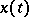of (1) defined for all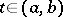with initial conditions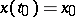,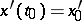.

If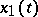and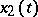are linearly independent solutions of the corresponding homogeneous equation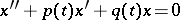(2)

and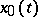is a particular solution of the inhomogeneous equation (1), then the general solution of (1) is given by the formula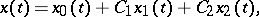where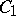and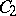are arbitrary constants. If one knows one non-zero solutionof (2), then a second solutionof it, linearly independent of, is given by the formula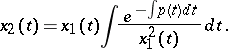If two linearly independent solutionsandof (2) are known, then a particular solutionof (1) can be found by the method of variation of constants.

In the study of (2) an important role is played by transformations of it to equations of other types. For example, by the change of variables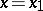,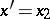, equation (2) reduces to a normal system of linear equations of the second order; by a change of the unknown function,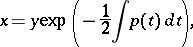equation (2) reduces to the equation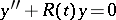, whereis called the invariant of equation (2); by the change of variable, equation (2) reduces to the Riccati equation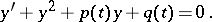After multiplication by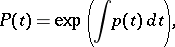equation (2) takes the self-adjoint formEquation (2) can be integrated by quadratures only in isolated cases. The most important special types of non-integrable equations (2) give rise to special functions.

Sturm's theorem on the separation of zeros: Ifandare linearly independent solutions of (2) and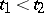are adjacent zeros of, then on the interval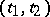there is exactly one zero of.

Suppose that in the equations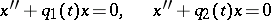(3)

the functionsandare continuous and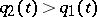on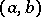. Then (the comparison theorem): Ifare adjacent zeros of a non-zero solution of the first equation in (3), then onthere is at least one zero of any solution of the second equation in (3).

The linear boundary value problem for equation (1) can be stated as follows: Find a solutionof (1) that satisfies the boundary conditions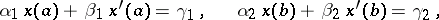where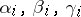are given constants and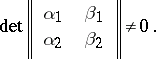The Sturm–Liouville problem for the equationwhere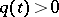is continuous on, can be stated as follows: Find those values of the parameterfor which this equation has a non-zero solutionsatisfying the boundary conditions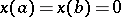. These values ofare called the eigen values, and the corresponding solutions are called the eigen functions.

Ifandin equation (2) are complex and the functionsandare holomorphic at the point, then for any numbersand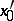there is a unique complex solutionof (2), holomorphic at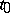, satisfying the initial conditions,. If in the equation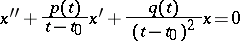(4)

the functionsand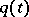are holomorphic at the pointand at least one of the numbers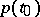,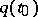,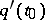is non-zero, thenis called a regular singular point for equation (4). In a neighbourhood of such a point one looks for a solution of (4) in the form of a generalized power series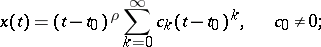(5)

hereis found from the defining equationwhereand. Suppose that the roots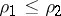of this equation are real. If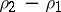is not an integer, then there are two linearly independent solutions of the form (5), for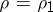and for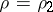. Ifis an integer, then, generally speaking, there is only one solution of the form (5), for; the second solution has a more complicated form (see ).